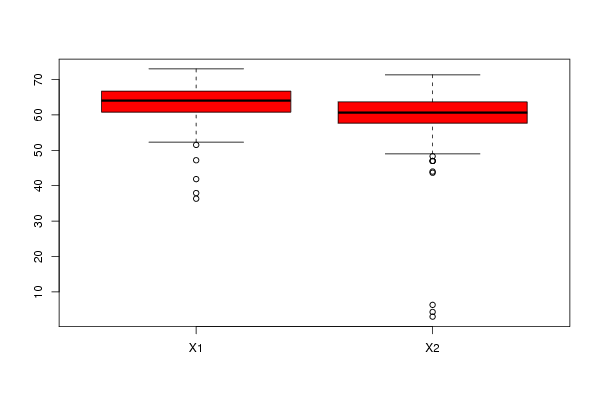## Free Statistics

of Irreproducible Research!

Author's title
Author*Unverified author*
R Software ModuleIan.Hollidayrwasp_Tests to Compare Two Means.wasp
Title produced by softwareAston University Statistical Software
Date of computationWed, 11 Nov 2009 11:51:00 +0100
Cite this page as followsStatistical Computations at FreeStatistics.org, Office for Research Development and Education, URL https://freestatistics.org/blog/index.php?v=date/2009/Nov/11/t1257943216pvan0zqa1smvd2s.htm/, Retrieved Sat, 30 Sep 2023 11:51:19 +0000
Statistical Computations at FreeStatistics.org, Office for Research Development and Education, URL https://freestatistics.org/blog/index.php?pk=55537, Retrieved Sat, 30 Sep 2023 11:51:19 +0000
QR Codes:Original text written by user:
IsPrivate?No (this computation is public)
User-defined keywordsPY2236 Aston University Language and Communication Module
Estimated Impact207
Family? (F = Feedback message, R = changed R code, M = changed R Module, P = changed Parameters, D = changed Data)
-     [Aston University Statistical Software] [Test of Two Means] [2009-11-10 17:16:17] [98fd0e87c3eb04e0cc2efde01dbafab6]
- R P   [Aston University Statistical Software] [Compare Two Means] [2009-11-11 08:09:40] [74be16979710d4c4e7c6647856088456]
-           [Aston University Statistical Software] [PY2236 Week 6 Dat...] [2009-11-11 10:51:00] [d41d8cd98f00b204e9800998ecf8427e] [Current]
Feedback Forum

Post a new message
Dataseries X:
70.8	66.67
69.6	66.33
69.87	64.33
67.47	64
67.6	63.33
67.13	61.33
66.27	64.67
66.73	63
68.07	60.67
67.8	63.67
64.8	60.67
64.6	61.67
64.2	62.33
64.2	60.33
63.67	59.67
61	60.33
59.67	59.33
59.67	58.67
59.8	58.67
60.73	59.33
59.4	57.33
58.07	59.33
57.47	56
70.73	53.67
72.87	58.67
66	49.33
66.07	71.33
66	70.33
66.27	69
64	66
63.67	66
63.73	63.33
63.33	65.33
63.53	64.33
63.53	64
62.87	61.67
59.53	63.67
62.8	64.67
60.8	61.67
59.8	62
56.67	61.33
57.67	63.67
58.4	61.33
55.47	62.33
56.2	59.67
71.53	59.33
68.67	61.67
65.67	58.67
66.73	58
67.33	56.67
66.73	59.67
66.87	58
65.8	57
64.73	57.67
65.47	58.67
63.6	55.33
64.07	56
64.67	55.67
63.73	53.33
62.53	53.67
61.93	51
62.67	47
62.8	4.33
61.33	70
62.6	68.67
59.13	67.67
61.27	66
59.47	65.67
57.87	65.67
59.73	63.67
61.4	63.67
58.8	64
58.33	62
57.47	62
57.13	61.67
55	61.67
51.53	63.33
72.73	61
73	62.33
70.8	60.33
70.07	60.33
71.67	60.67
71.07	57.67
70.67	58.33
70.73	58
70.73	57.33
68.6	56.67
69.6	58
66.47	55.33
67.07	55.67
68.67	54.67
66.93	56.33
65.93	55
68.87	55
66.53	54.67
65.8	54.33
66.6	49
66	48.33
65	49.67
66.8	43.67
65.6	6.33
66	3
65.67	66.67
64.67	67.33
65.07	65.33
64.67	66
65.07	65.67
65.2	66.67
64.87	65.67
63.47	65
62.6	64.67
64.07	66.67
63.73	63.67
64.67	63.33
61.6	63.67
61.6	63.33
60.47	63.67
61.27	63
63	61.67
61.47	61.33
60.87	60.67
61.67	60
62.87	61.67
62.4	61.33
59.73	58.67
60.13	60.33
58.8	59.67
59.6	59.33
58.93	59.67
60.13	61
58.2	61
58.27	60
58.27	60
55.07	58.67
53.87	58.33
52.33	58
47.2	56.33
37.93	54.67
72.73	55.33
70.07	54
70.67	52.67
72.07	44
68.8	65.67
68.8	65
67.47	66.33
66.73	64
66.53	62.33
66	61.33
67.6	63
66	63.67
66	62
66.53	61.33
65.8	64.67
64.27	62.67
64.67	64
64.6	61
64.13	60.67
65.47	59.67
62.93	60.33
63.53	56.67
62.13	56.67
63.87	54.33
64.67	51
63.33	51
63.13	47
62.8	68
62.4	65
62.4	64
62.6	64
61.47	64
62.2	62
63	61
61.8	60
59.73	60
60.33	62
60.13	60
59.53	59
59	61
55.93	60
41.87	60
36.33	58
71.67	58
71.47	60
70.47	58
69.53	59
70.73	56
69.93	54
68.73	51
67.53	47


 Summary of computational transaction Raw Input view raw input (R code) Raw Output view raw output of R engine Computing time 2 seconds R Server 'George Udny Yule' @ 72.249.76.132

\begin{tabular}{lllllllll}
\hline
Summary of computational transaction \tabularnewline
Raw Input & view raw input (R code)  \tabularnewline
Raw Output & view raw output of R engine  \tabularnewline
Computing time & 2 seconds \tabularnewline
R Server & 'George Udny Yule' @ 72.249.76.132 \tabularnewline
\hline
\end{tabular}
%Source: https://freestatistics.org/blog/index.php?pk=55537&T=0

[TABLE]
[ROW][C]Summary of computational transaction[/C][/ROW]
[ROW][C]Raw Input[/C][C]view raw input (R code) [/C][/ROW]
[ROW][C]Raw Output[/C][C]view raw output of R engine [/C][/ROW]
[ROW][C]Computing time[/C][C]2 seconds[/C][/ROW]
[ROW][C]R Server[/C][C]'George Udny Yule' @ 72.249.76.132[/C][/ROW]
[/TABLE]
Source: https://freestatistics.org/blog/index.php?pk=55537&T=0

Globally Unique Identifier (entire table): ba.freestatistics.org/blog/index.php?pk=55537&T=0

As an alternative you can also use a QR Code:

The GUIDs for individual cells are displayed in the table below:

 Summary of computational transaction Raw Input view raw input (R code) Raw Output view raw output of R engine Computing time 2 seconds R Server 'George Udny Yule' @ 72.249.76.132

 Students T-Test Statistic P-value Students T-Test 5.60536 0

\begin{tabular}{lllllllll}
\hline
Students T-Test \tabularnewline
& Statistic & P-value \tabularnewline
Students T-Test & 5.60536 & 0 \tabularnewline
\hline
\end{tabular}
%Source: https://freestatistics.org/blog/index.php?pk=55537&T=1

[TABLE]
[ROW][C]Students T-Test[/C][/ROW]
[ROW][C][/C][C]Statistic[/C][C]P-value[/C][/ROW]
[ROW][C]Students T-Test[/C][C]5.60536[/C][C]0[/C][/ROW]
[/TABLE]
Source: https://freestatistics.org/blog/index.php?pk=55537&T=1

Globally Unique Identifier (entire table): ba.freestatistics.org/blog/index.php?pk=55537&T=1

As an alternative you can also use a QR Code:

The GUIDs for individual cells are displayed in the table below:

 Students T-Test Statistic P-value Students T-Test 5.60536 0PNG link Postscript link PDF link

Parameters (Session):
par1 = two.sided ; par2 = 1 ; par3 = 2 ; par4 = Students T-Test ; par5 = paired ; par6 = 0.0 ;
Parameters (R input):
par1 = two.sided ; par2 = 1 ; par3 = 2 ; par4 = Students T-Test ; par5 = paired ; par6 = 0.0 ; par7 = ; par8 = ; par9 = ; par10 = ; par11 = ; par12 = ; par13 = ; par14 = ; par15 = ; par16 = ; par17 = ; par18 = ; par19 = ; par20 = ;
R code (references can be found in the software module):
par2 <- as.numeric(par2)par3 <- as.numeric(par3)par4 <- as.character(par4)par5 <- as.character(par5)par6 <- as.numeric(par6)if ( par5 == 'unpaired') paired <- FALSE else paired <- TRUEx <- t(y)bitmap(file='test1.png')(r<-boxplot(x ,xlab=xlab,ylab=ylab,main=main,notch=FALSE,col=2))dev.off()load(file='createtable')if( par4 == 'Wilcoxon-Mann_Whitney'){a<-table.start()a <- table.row.start(a)a <- table.element(a,'Wilcoxon Test',3,TRUE)a <- table.row.end(a)a <- table.row.start(a)a <- table.element(a,'',1,TRUE)a <- table.element(a,'Statistic',1,TRUE)a <- table.element(a,'P-value',1,TRUE)a <- table.row.end(a)W <- wilcox.test(x[,par2],x[,par3],alternative=par1, paired = paired)a<-table.row.start(a)a<-table.element(a,'Wilcoxon Test',1,TRUE)a<-table.element(a,W$statistic[])a<-table.element(a,round(W$p.value, digits=5) )a<-table.row.end(a)a<-table.end(a)table.save(a,file='mytable.tab')}if( par4 == 'Students T-Test'){a<-table.start()a <- table.row.start(a)a <- table.element(a,'Students T-Test',3,TRUE)a <- table.row.end(a)a <- table.row.start(a)a <- table.element(a,'',1,TRUE)a <- table.element(a,'Statistic',1,TRUE)a <- table.element(a,'P-value',1,TRUE)a <- table.row.end(a)T <- t.test(x[,par2],x[,par3],alternative=par1, paired=paired, mu=par6)a<-table.row.start(a)a<-table.element(a,'Students T-Test',1,TRUE)a<-table.element(a,round(T$statistic[], digits=5) )a<-table.element(a,round(T$p.value, digits=5) )a<-table.row.end(a)a<-table.end(a)table.save(a,file='mytable.tab')}